# A Chess Puzzle, Part V: Rooks and TopologyPart V of A Mathematical Chess Puzzle:

### Review

We — that is, I — have been studying the (classic) “unguarded” chess puzzle, which is to say, how to pack as many of one chess piece on a chess board without any of the pieces attacking any others. But with the twist, that the board may be infinite, and possibly uncountably infinite. In the last post we saw that the set of maximal unguarded solutions for the Rook on the board $B_A$ (for any set $A$) had an interesting mathematical property, that they could be represented as the permutation group on the set $A$. In later posts we will see that the Queens also have some interesting properties, some of which can already be seen in our current focus on the Rook.

### What is Topology and Why does it Matter?

The glib definition of topology is “rubber sheet geometry”, which is to say, the study of what properties are preserved in an object even if they are made of rubber and can be twisted and stretched but not “torn”. For example, a solid ball and a solid cube can be deformed into each other just by stretching, but to deform a ball into a 1-holed doughnut you would need to puncture the ball to make the hole — which is not allowed.

One way in which a “topology” can be defined on a space is by specifying the way in which two points are “close” to each other, or whether two points can be “connected” by a continuous path.

We have been defining “chess boards” as two-dimensional things (denoted $B_A$) whose “squares” are identified with coordinates (x,y), where the x and y are in some subset $A$ of the real line $\mathbb{R}$. For example, the standard 8×8 chessboard we called $B_8$ uses the coordinates in the subset $\mathbb{Z}_8 = \{ 0, 1, 2, … 7 \}$ (though we could just as easily have taken {1, … 8} ). Among the other coordinate sets $A$ we could consider are the whole numbers, the rationals, the algebraic numbers, and the non-negative real numbers, or for that matter the whole real line.

### Topological Density

To see why questions about chess puzzles on various boards $B_A$ may have different answers depending on topology, consider that one of the properties of a subset of $\mathbb{R}$ is called density. A set $A \subseteq \mathbb{R}$ is said to be dense at a point $x \in A$ if for any other point $y \in A$ where $y \neq x$, there exists another point $z \in A$ strictly between them, ie, such that $(z-x)*(z-y) < 0$. The set A is everywhere dense if it is dense at every point of A, and nowhere dense (also called “discrete”) if it is not dense at any point of A.

For example, the whole numbers $\mathbb{W}$ is nowhere dense because there are no other integers between n and n+1. Meanwhile, the rationals $\mathbb{Q}$ are dense, because if $p$ and $q$ are two distinct rational numbers (ie, can be expressed as an integer fraction $m/n$), then it can be shown that $(p+q)/2$ is another rational strictly between them.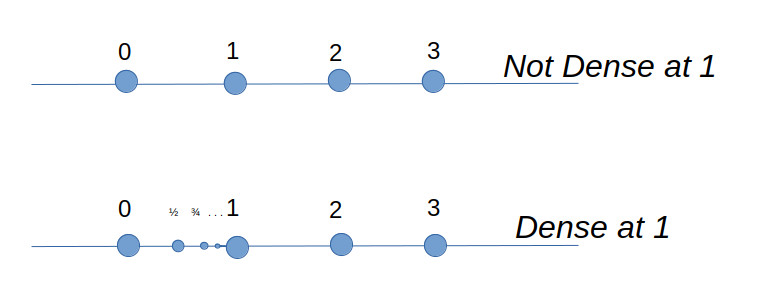And so, to see the reason why density is relevant for chess, simply note that the moves and attack regions for both the King, Pawn and Knight are defined by the squares *adjacent* to their current location, and on a board $B_A$ where $A$ is everywhere dense, there is no such thing as an adjacent square, because there is always another square in A in-between. In other words, the “unguarded” puzzle for Kings, Pawns and Knights does not make sense for dense sets $A$ such as rationals, algebraics, or reals. Meanwhile, because the attacks of Bishops, Rooks, and Queens is defined in terms of directions on the board (horizontal, vertical, diagonal), they have well-defined meanings even on everywhere dense boards.

### Connectedness

Strictly speaking, a set is topologically connected if it cannot be represented by a union of two disjoint sets. By that definition, a set like the integers or the rationals is nowhere connected. For example, the rationals can be represented as $\mathbb{Q} = \mathbb{Q}^+ \cup \mathbb{Q}^-$, where $\mathbb{Q}^+$ and $\mathbb{Q}^-$ are the sets of rationals greater and less than $\sqrt{2}$, respectively. These are both open sets because for any rational number $x \in \mathbb{Q}^+$, all other rationals (sufficiently close) to $x$ are also in the same set.

Nevertheless, we can still define “weaker” forms of connectedness on the rationals, in the sense that the closure of $\mathbb{Q} \subset \mathbb{R}$ is connected. There are also other more general definitions. A set is considered connected, intuitively, if you can get from any place in the set to any other by a “connected” path lying wholly within the set (whose definition needs to be made clear).

For example, suppose we take the set of whole numbers $\mathbb{W}$, and define that two elements $m, n \in \mathbb{W}$ to be “weakly connected” if they differ by no more than 1. So, 2 is connected to 3 and 1, as well as itself, but 2 is not connected to 4. More generally, we can say that two subsets A and B of $\mathbb{W}$ are weakly connected to each other, if there exists a member a in A and b in B such that a and b are connected. Finally, we can define a single subset $A \subseteq \mathbb{W}$ to be weakly connected, if for any two elements $a,b \in A$, there exists a finite sequence of elements $\{ a_i \} \subset A$ such that $a = a_0, a_n = b$, and where $a_i$ and $a_{i+1}$ are connected for all $i = 0…n-1$.

With this definition, we can see that the set $A = \{0,1,2,3,4\}$ is a weakly connected set, but that $A=\{0,1,3,4\}$ is not connected, because there is no way to to get from 1 to 3 by connected elements in $A$ (2 is missing and forms a gap).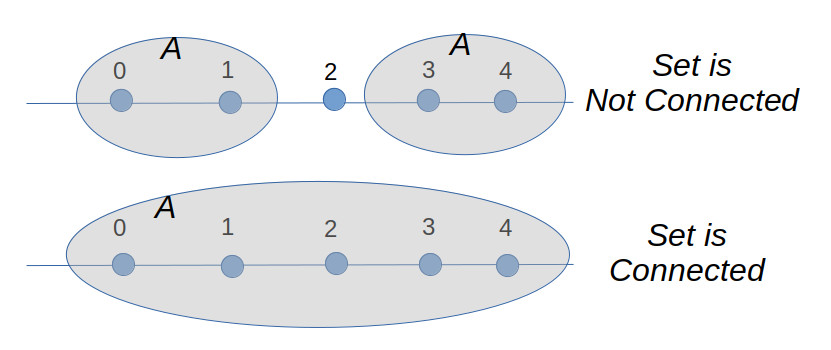The reason all this matters is that we will see that for the solutions to the unguarded rook problem, the topology of those solutions differs when we go from the infinite integer board $B_{\omega}$ to the infinite rational board $B_{\mathbb{Q^{+}}}$ or real board.

### The Topology of the Integer Board

Just as with the rationals, we can define “weakly connected” on the integer board $B_{\mathbb{W}}$, by first specifying that two squares a and b are “weakly connected” if they are a Kings move away from each other, that is, one square away horizontally, vertically, or diagonally. And by extension, we can say that any subset $A$ of $B_{\mathbb{W}}$, is connected, if any two members $a$ and $b$ of $A$ can reach each other, by a king moving strictly within the set $A$. From this point on, we will use “connected” to mean “weakly connected”, unless we say otherwise.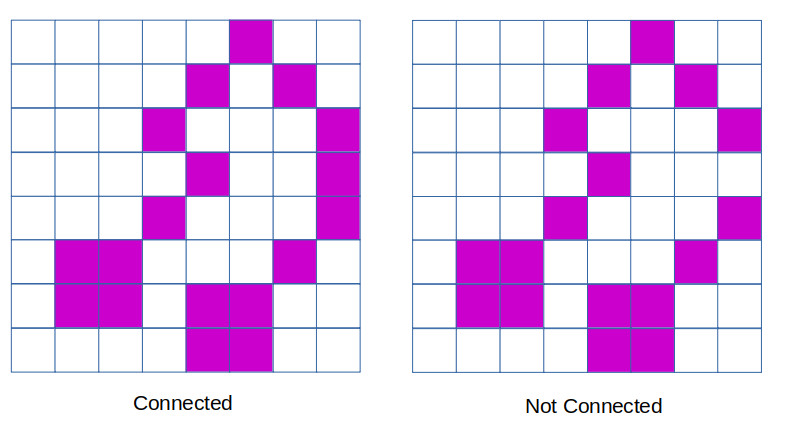Connected Rook Theorem 1: On any finite board $B_n$, the only connected maximal solutions to the unguarded rook problem are the two diagonal solutions, ie the graphs of the function $y=\phi(x)$, where

$$\phi(x) = x$$

or

$$\phi(x) = n – 1 -x$$

We first claim that if two squares in an unguarded rook solution are both connected to a third one, then all three must lie on the same diagonal (northwest or northeast). For, consider the case where square $a$ is connected to square $b$ along the north-east diagonal. We can go through and eliminate with an x all of the squares which are attacked by either $a$ or $b$. And what we see is that the only remaining square which a third rook can be placed and still be attached to $a$ is the lower-left corner.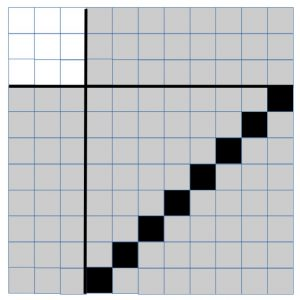By repeated application of this claim, what we have just shown is that all of the rooks in a connected component of an unguarded rook solution must lie on the same diagonal. Since this is a maximal unguarded solution, there must be a rook on the first row somewhere. We now claim that it must either be on the leftmost square (forming the northeastern diagonal solution), or else the rightmost square (forming the northwestern diagonal solution). For suppose that the rook is anywhere else along the bottom row, and that (without loss of generality) that it is part of a northeasterly diagonal.

Once again, by a process of eliminating all other squares under attack by the northeasterly diagonal line of rooks, we can see that this leaves a disconnected square in the upper left corner as the only available locations of the rooks that must occupy the remaining rows and columns. But none of these squares are connected to the northeast diagonal, and so a maximal unguarded solution cannot be constructed in this manner that is also connected.

QED

Connected Rook Theorem 2: On the infinite integer board $B_{\omega}$, the only connected maximal solution to the unguarded rook problem is the main diagonal solution, $\phi(x) = x$.

Proof: Clearly the above solution is maximal. Suppose that there is another maximal solution where there is a rook located at a position $P = (m,n)$, where (without loss of generality) we can assume $m > n > 0$. By eliminating all cells attacked by this rook, we now have a board segmented into four non-empty regions, (I, II, III, IV),  each of which would be disconnected from each of the others, save for their connection to the rook at $P$.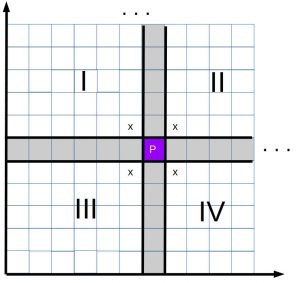By the same argument previously, either $P$ connects regions I to IV by the north-west diagonals, or else it connects regions II and III, by the northeast diagonals. If we choose to join regions II and III, then region III (including P) is an $m x n$ rectangle, in which we would have to fit $m$ rooks, even though we only have $n$ rows. This would force at least two rooks to occupy the same rows, which is not allowed. This leaves us with P joining up regions I and IV, by a northwest diagonal.  Now region I only has $m-1$ columns, while region IV has only $n-1$ rows, and so we have only a finite number of additional rooks we can add to this solution (region II not being connected to P). Thus neither option allows us to define a maximal solution to the unguarded rook problem, meaning that we must conclude that only the case where $m=n$ is valid.

QED.

### Unguarded Rooks on a Rational Board

While we could take the entire set $\mathbb{Q}$ of rational numbers, for our purposes it suffices to look at a much smaller but equivalent space, which is the unit interval of rational numbers, ie

$$\mathbb{Q}^* = \mathbb{Q} \cap [0,1]$$

The set of rationals is countably infinite, and so our board $B_{\mathbb{Q}^*}$ has the same number of “squares” as the infinite integer board. Nevertheless, the unguarded rook problem on this board has a surprising answer in store for us, given by the following theorem:

Connected Rook Theorem 3: There are an infinite number of connected maximal unguarded Rook solutions on the rational unit board $B_{\mathbb{Q}^*}$.

Comment: Just so you can see how on earth this could be possible, consider just one valid solution, defined on $\mathbb{Q}^*$ by the function:

$$\phi(x)= \begin{cases} \frac{x}{2}, & \text{if x \in [0, \frac{2}{3})} \\ 2x – 1, & \text{x \in [\frac{2}{3}, 1]} \end{cases}$$

The function $\phi(x)$ is a piecewise-linear function, which is a bijection on the set $\mathbb{Q}^*$.

The graph on $B_{\mathbb{Q}^*}$ shows that the placement of the rooks on the board looks like this: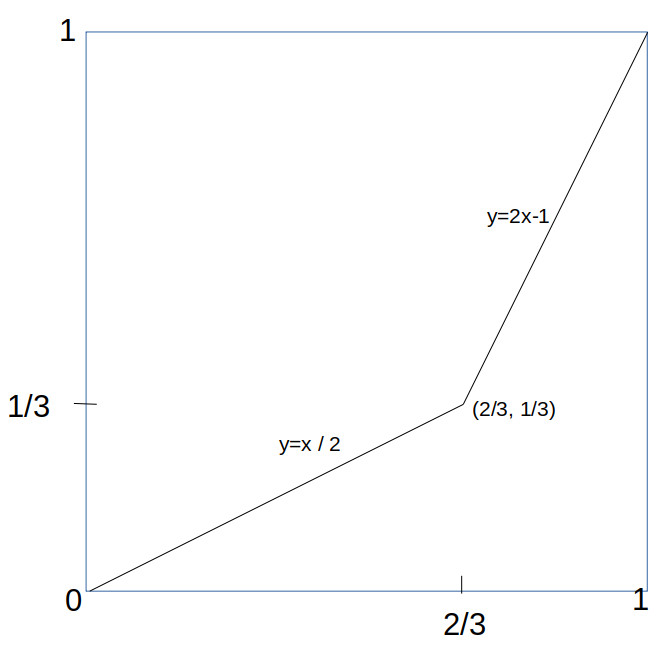what makes this work is that linear functions are invertible on the rational numbers, and that the rational numbers (unlike the integers) are *dense*. The same trick would not work on the integers, because you can’t apply y = x/2 to the odd integers and get another integer back.

Just to remind the reader of what this picture is about, that thin line is a chess position, consisting of a set of infinitely many rooks along that line, so an (infinite) magnification would reveal them, like this: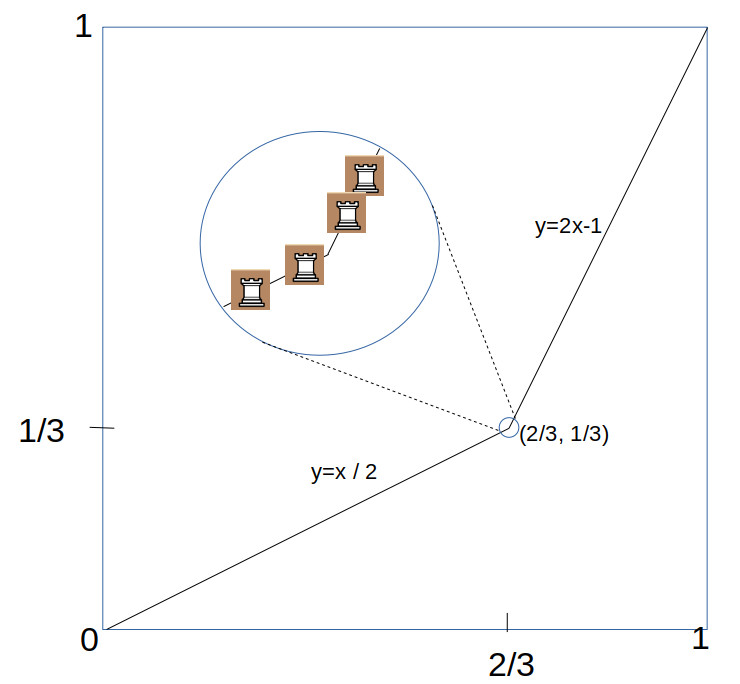This example is just one of many other such piecewise-linear functions which do the trick. The only requirement is that the functions must be strictly monotonic (increasing or descreasing) on the interval $\mathbb{Q}^*$, and (to ensure it is maximal), covers the entire interval, either by $\phi(0) = 0, \phi(1) = 1$ or else $\phi(0) = 1, \phi(1) = 0$.

It turns out that the number of such functions is infinite. This can be seen easily by just noting that there was nothing special about the bend at $x=2/3$, and you could create a similar function with a bend on any other rational number between zero and one, of which there are countably infinite many of them.

### Fractal Content

The (two-dimensional) density of the Rook solutions is zero, since lines have zero area. This is the case for all three pieces Rook, Bishop and Queen. But we can still compare the relative density of each of these pieces, by measuring the (one-dimensional) “fractal” content of the positions. For example, the fractal content of the solution shown above is simply the length of the two line segments, which is $2\sqrt{5}/3 \approx 0.75$. But we can actually do much better, by pushing the “corner” of the two lines toward the bottom right like this: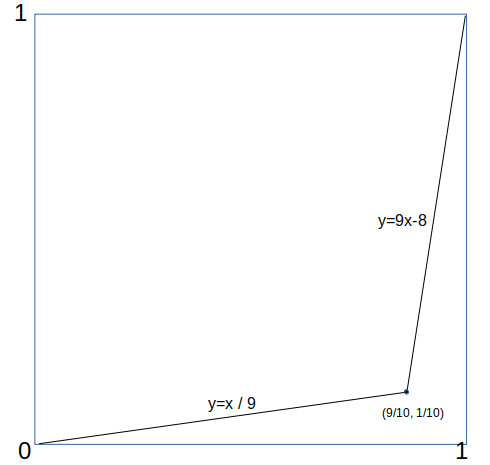in which case the fractal density is $\sqrt{82}/10 \approx 1.82$. As can be seen, by pushing further the rook solutions can attain fractal densities as close to 2 as we like.

### Uncountably Many Solutions

We showed above that there are an infinite number of connected maximal Rook solutions on the rational interval board $B_{\mathbb{Q}^*}$. But you can actually say a lot more; in fact the number of such solutions can be shown to be uncountably infinite, which is to say, there are at least as many of these solutions as there are real numbers (the infinity of the continuum) !

One way you can prove that the number of solutions is uncountable, is to construct a mapping between the (uncountable) set of all infinite decimals between 0 and 1, and a piecewise-linear function with rational coefficients, whose graph is a maximal connected solution of the unguarded Rook problem.

To do this, we first define for each digit from $i = 0 . . . 9$, a different piecewise linear function $f_i()$ on the unit square, simply by mapping the digits to different “bend locations” in the square (having rational coordinates):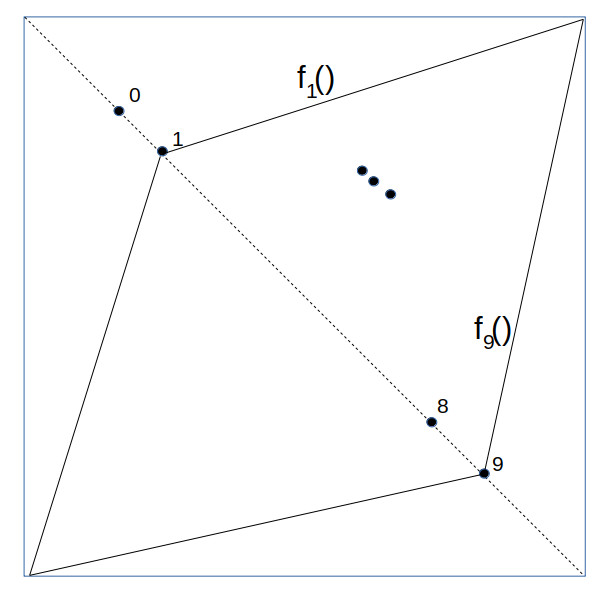Next, we take the unit square and along the main diagonal, lay out an infinite number of smaller squares, each half the size of the previous: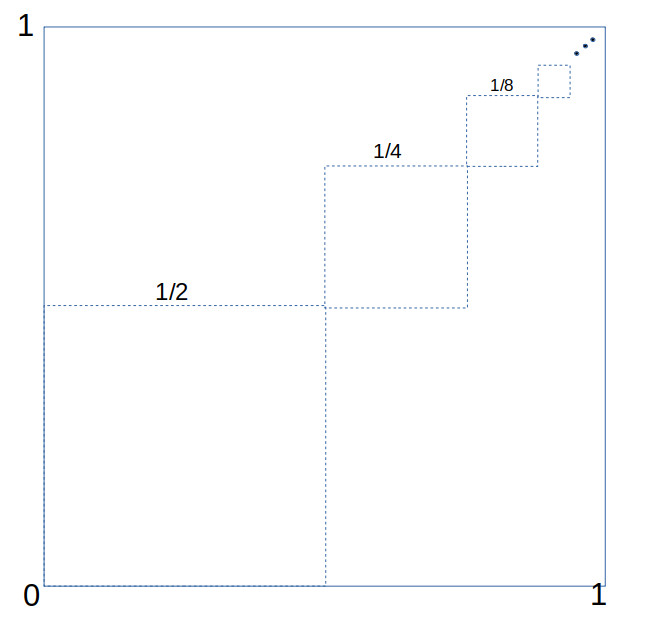We now show how to map each infinite decimal fraction $z$ (e.g. $z = 0.2739 . . .$ ) to a specific piecewise-linear function $F = F_z(x)$ on the unit square. For each of the smaller squares we have placed inside the unit square, we go through the decimal digits in $z$ and for each digit $d$ define the corresponding function $F_z(x)$ restricted to that range by $F_z(x) = f_d(x)$, corresponding to that digit $d$.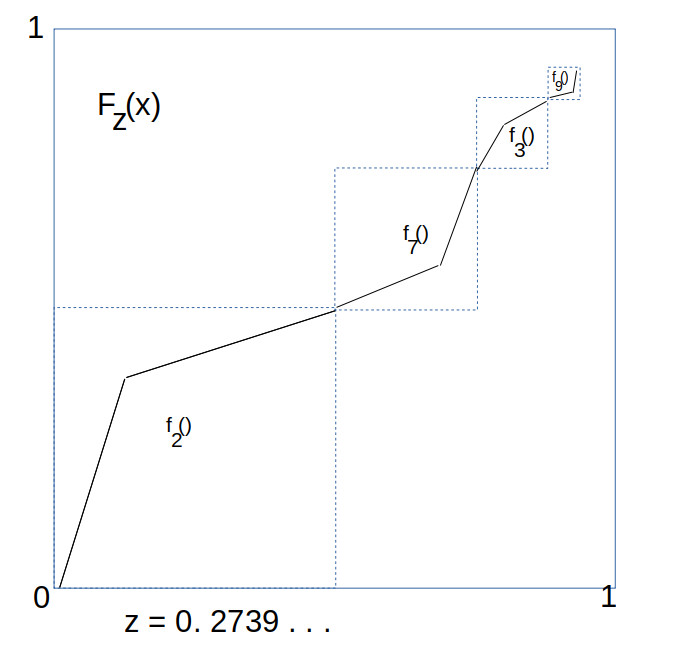As these infinite number of squares converge to the point $(1,1)$, we define $F_z(1) = 1$, which gives a complete definition on the whole unit interval. Since this mapping is injective, this means that the number of piecewise linear functions with rational coefficients is at least as large as the number of reals on the unit interval, which is uncountably infinite. This completes the proof.

So yes, there are a lot of them. And again, the reason this is so different from the integers has to do with the topological density of the sets.

Just to close the loop, if we look at the full real interval $I = [0,1] \subset \mathbb{R}$, it is actually much easier to construct topologically connected (in fact, compact) maximal unguarded Rook solutions. To construct such solutions, all that we require is that it be the graph of a function $y = \phi(x)$, where $\phi$ is a bijection on $[0,1]$ such that it is continuous and monotonically increasing or decreasing. That is, if $x > y$ then $\phi(x) > \phi(y)$. A simple set of examples of this are the powers of x, such as $\phi(x) = x^2, \phi(x) = x^3$, and so on.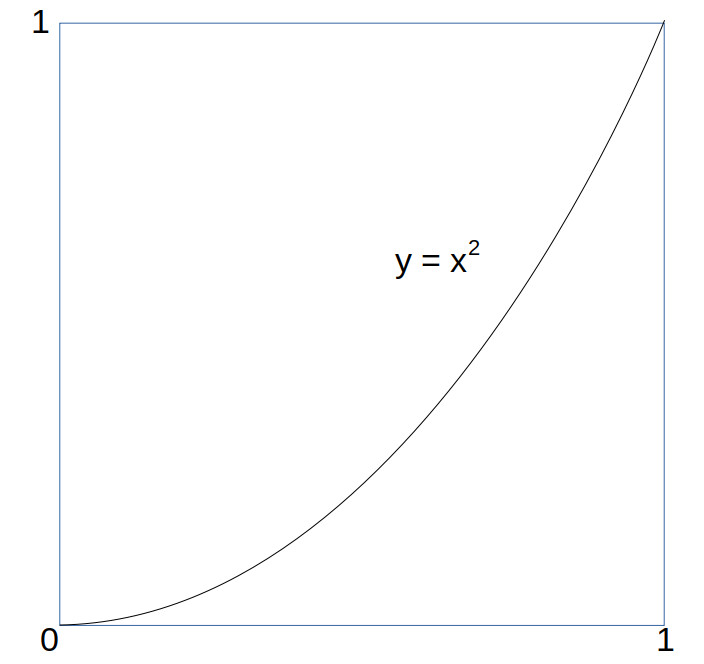As with the rational board case, with higher powers of x the curve begins to hug the bottom and right side of the board, with a curve length approaching 2. But can we do any better, or is 2 the largest 1 dimensional fractal content we can attain for connected unguarded rook solutions? The answer is given by the following theorem:

Fractal Content Rook Theorem: The path length of  a connected maximal unguarded Rook solution on the unit interval is not more than 2. That is, the length of any curve $y = \phi(x)$ on the unit interval $[0,1]$ where $\phi(x)$ is a continuous monotonically increasing function from 0 to 1 on the interval is less than or equal to 2.

Proof: We will need to use a little bit of calculus to do this. First, we parameterize the curve by $c(t) = (x(t),y(t))$ where the parameter $t$ ranges from 0 to 1, and

$$\begin{cases} x = x(t) = t \\ y = y(t) = \phi(x(t)) \end{cases}$$

From this we can compute the path length $s$ given by the integral (ie, sum):

$$s = \int_{t=0}^1 \frac{ds}{dt} dt$$

where $ds$ is the incremental increases in the path distance by $(dx,dy)$, given by

$$ds = \sqrt{dx^2 + dy^2}$$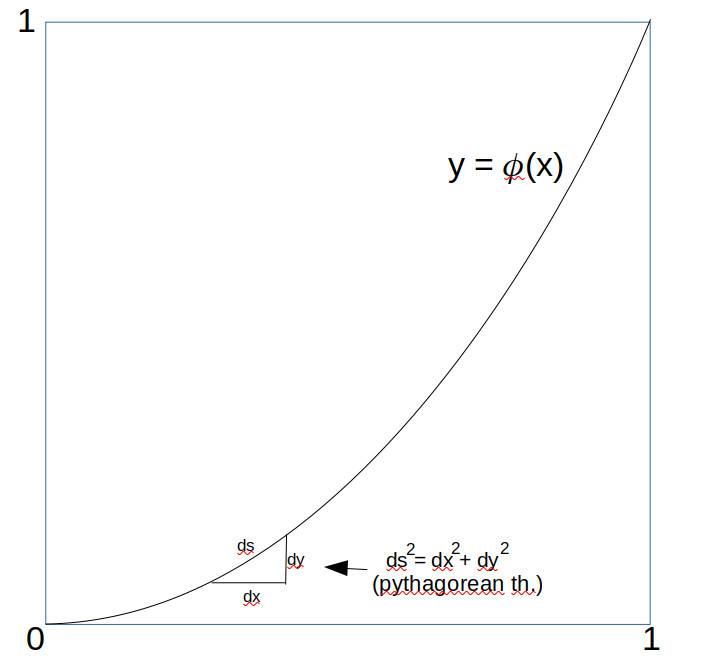For those unversed in calculus, think of $ds$ as just very small line segments into which the curve has been broken, and use Pythagorean Theorem). Now $dx/dt = 1$ since $x=t$, and $dy/dt \ge 0$, given that $y = \phi(x)$ is monotonically increasing. We can thus take advantage of the inequality

$$\sqrt{a^2 + b^2} \le \sqrt{a^2 +2ab + b^2} = (a + b)$$

when $a,b \ge 0$ to assert that (formally)

$$\frac{ds}{dt} = \sqrt{(\frac{dx}{dt})^2 + (\frac{dy}{dt})^2} \le \sqrt{(\frac{dx}{dt} + \frac{dy}{dt})^2} = \frac{dx}{dt} + \frac{dy}{dt}$$

and so we can conclude (since x(1) = 1 and y(1) = 1):

$$s \le \int_{t=0}^1 \frac{dx}{dt} + \int_{t=0}^1\frac{dy}{dt}dt = 1 + 1 = 2$$

which was to be proved. QED

We have now pretty much exhausted the study of unguarded Rooks, so it’s time to touch base briefly on the Bishop, before we move on (at last) to the unguarded Queens.